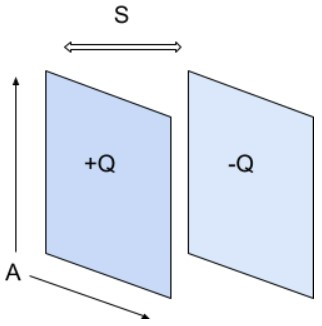# Capacitance Calculator

Enter the values in the boxes below to calculate capacitance between parallel plate capacitors by using the capacitance calculator.

F/m
m2
m

Give Us Feedback

The capacitor calculator is an online tool that is used to calculate capacitance by taking permittivity, the distance between plates, and the area of plates as input.

## What is a capacitor?

A capacitor is a device that can store electric charges. The most common type of capacitor, shown below, is a parallel capacitor. In it type of capacitor, the plates of a capacitor are separated by an insulator.### Capacitance

Capacitance is the amount of electric charge which a capacitor can store.

## Capacitance formula

The formula used to find capacitance is

C = ε A/S

In this equation:

A = the area on which plates overlap

S = distance between plates

ε = the permittivity of the conductor

The value of permittivity varies from conductor to conductor. To find capacitance with charge and potential difference, use our other capacitance calculator.

## How to calculate capacitance?

Example:

Calculate the capacitance of a capacitor that has 0.03 m2 area of its plates separated by free space and the distance between the plates is 0.7 m.

Solution:

Step 1: Identify values.

A = 0.03 m2

S = 0.7 m

Permittivity of free space = 8.85418782 × 10-12 m-3 kg-1 s4 A2

Step 2: Put the values in the capacitance formula.

C = ε A/S

= 8.85418782 × 10-12 ×0.03 / 0.7

= 0.38 F

### Math Tools